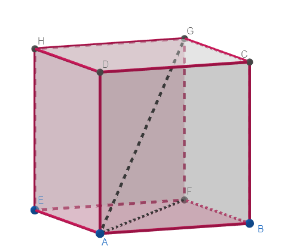The length of the longest rod that can fit in a cubical vessel of side 10cm is  \begin{align} & \left( A \right)\text{ }10cm \\ & \left( B \right)\text{ }10\sqrt{2}cm \\ & \left( C \right)\text{ }10\sqrt{3}cm \\ & \left( D \right)\text{ }20cm \\ \end{align}Verified
146.1k+ views
Hint: We solve the given question by noticing the fact that distance between any two points on the cube is maximum when they are the endpoints of the diagonal of the cube. So, we start by finding the length of the diagonal of any face using Pythagoras theorem and then we select another side of the cube such that they form a right-angled triangle and use the Pythagoras theorem again to find the length of the diagonal.

We were given that the length of the side of the cube is 10cm.
We need to find the length of the longest rod that has to be fitted in the cube. As the rod has to touch the cube at two points, it means we have to find the longest distance between any two points on the sides of the cube.
Longest distance between two points of the cube is when it is the diagonal of the cube.Let the length of the side of the cube be $a=10cm$ .
The longest distance is the diagonal of the cube which is AG from the above figure.
First, let us find the length of the side AF which is the diagonal of the face ABFE.
As ABFE is the face of a cube, it is square with side length $a=10cm$ . Then $\Delta ABF$ is a right-angled triangle.
So, by using the Pythagoras theorem, which is
If a, b are adjacent sides of a right-angled triangle and c is the hypotenuse, then
${{a}^{2}}+{{b}^{2}}={{c}^{2}}$ .
So, by applying the Pythagoras Theorem on the $\Delta ABF$ , we get
\begin{align} & \Rightarrow \text{ }{{\left( AB \right)}^{2}}+{{\left( BF \right)}^{2}}={{\left( AF \right)}^{2}} \\ & \Rightarrow \text{ }{{\left( a \right)}^{2}}+{{\left( a \right)}^{2}}={{\left( AF \right)}^{2}} \\ & \Rightarrow \text{ }{{\left( AF \right)}^{2}}=2{{a}^{2}} \\ & \Rightarrow \text{ }AF=\sqrt{2}a \\ \end{align}
Now consider the $\Delta AFG$ , where $AF=\sqrt{2}a$ , $FG=a$ as it is a side of the cube and $\angle F={{90}^{\circ }}$ .
So, by applying the Pythagoras Theorem again on the $\Delta ABF$ , we get
\begin{align} & \Rightarrow \text{ }{{\left( AF \right)}^{2}}+{{\left( FG \right)}^{2}}={{\left( AG \right)}^{2}} \\ & \Rightarrow \text{ }{{\left( a \right)}^{2}}+{{\left( \sqrt{2}a \right)}^{2}}={{\left( AG \right)}^{2}} \\ & \Rightarrow \text{ }{{\left( AG \right)}^{2}}={{a}^{2}}+2{{a}^{2}} \\ & \Rightarrow \text{ }{{\left( AG \right)}^{2}}=3{{a}^{2}} \\ & \Rightarrow \text{ }AG=\sqrt{3}a \\ \end{align}
As $a=10cm$ , by substituting it in the above obtained answer, we get
\begin{align} & \Rightarrow \text{ }AG=\sqrt{3}a \\ & \Rightarrow \text{ }AG=\sqrt{3}\times 10 \\ & \Rightarrow \text{ }AG=10\sqrt{3}cms \\ \end{align}
So, we get that the length of the diagonal of the cube is equal to $10\sqrt{3}cms$ .
So, the length of the longest rod that can fit in a cube of side 10cms is $10\sqrt{3}cms$ .
So, the correct answer is “Option C”.

Note: There is a possibility of one making a mistake by considering the diagonal of a face of the cube as the diagonal of the cube, then the answer obtained will be $10\sqrt{2}cms$ , but it is wrong as the longest distance between two points in a cube is the diagonal between opposite edges of the cube not between that of the edges of the face of the cube.